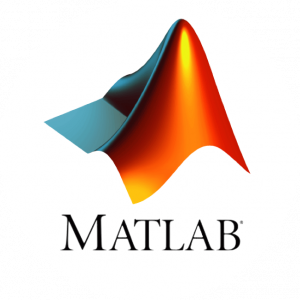# Take part in “Introduction to MATLAB” (Part 2)In order to develop skills with MATLAB software and use them for the work with MATLAB in the future, we invite you to take part in MATLAB course “Introduction to MATLAB” (Apart 2). Prerequisites – knowledge in MATLAB, for example, attendance of “Introduction to MATLAB” (Part 1).

The course will start on October 31.

MATLAB training will take place  online, every Monday from 16:30 to 18:00. Duration- 8 lessons.

“Introduction to MATLAB” (Part 2) offers an opportunity to continue learning the basics of MATLAB software. The current course is made as a continuation to the course “Introduction to MATLAB” (Part 1). Participants will continue to learn the high-programming language MATLAB – elementary
math functions, linear algebra functions, as well as graphing questions. Optimization issues will be considered: minimization, in the case of one, several variable functions, replacement by an orthogonal series of functions and an approach to formulating the optimization problem. Basic issues of solving differential equations will also be covered, as well as creating applications with the app builder and calculating using parallel computing.

More information on “Introduction to MATLAB” (Part 2) and registration is available here.